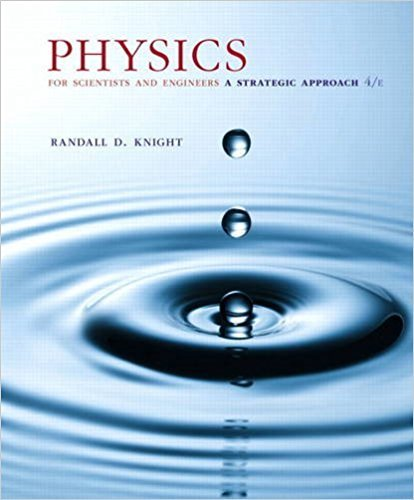×
×

# FIGURE CP35.50 shows a simple zoom lens in which theISBN: 9780134081496 191

## Solution for problem 35.79 Chapter 35

Physics for Scientists and Engineers: A Strategic Approach, Standard Edition (Chs 1-36) | 4th Edition

• Textbook Solutions
• 2901 Step-by-step solutions solved by professors and subject experts
• Get 24/7 help from StudySoup virtual teaching assistantsPhysics for Scientists and Engineers: A Strategic Approach, Standard Edition (Chs 1-36) | 4th Edition

4 5 1 265 Reviews
19
5
Problem 35.79

FIGURE CP35.50 shows a simple zoom lens in which the magnitudes of both focal lengths are f. If the spacing d 6 f, the image of the converging lens falls on the right side of the diverging lens. Our procedure of letting the image of the first lens act as the object of the second lens will continue to work in this case if we use a negative object distance for the second lens. This is called a virtual object. Consider an object very far to the left 1s 2 of the converging lens. Define the effective focal length as the distance from the midpoint between the lenses to the final image. a. Show that the effective focal length is feff = f 2 - fd + 1 2 d2 d b. What is the zoom for a lens that can be adjusted from d = 1 2 f to d = 1 4 f ?

Step-by-Step Solution:
Step 1 of 3
Step 2 of 3

Step 3 of 3

##### ISBN: 9780134081496

Physics for Scientists and Engineers: A Strategic Approach, Standard Edition (Chs 1-36) was written by and is associated to the ISBN: 9780134081496. The full step-by-step solution to problem: 35.79 from chapter: 35 was answered by , our top Physics solution expert on 12/28/17, 08:06PM. Since the solution to 35.79 from 35 chapter was answered, more than 227 students have viewed the full step-by-step answer. The answer to “FIGURE CP35.50 shows a simple zoom lens in which the magnitudes of both focal lengths are f. If the spacing d 6 f, the image of the converging lens falls on the right side of the diverging lens. Our procedure of letting the image of the first lens act as the object of the second lens will continue to work in this case if we use a negative object distance for the second lens. This is called a virtual object. Consider an object very far to the left 1s 2 of the converging lens. Define the effective focal length as the distance from the midpoint between the lenses to the final image. a. Show that the effective focal length is feff = f 2 - fd + 1 2 d2 d b. What is the zoom for a lens that can be adjusted from d = 1 2 f to d = 1 4 f ?” is broken down into a number of easy to follow steps, and 156 words. This textbook survival guide was created for the textbook: Physics for Scientists and Engineers: A Strategic Approach, Standard Edition (Chs 1-36), edition: 4. This full solution covers the following key subjects: . This expansive textbook survival guide covers 42 chapters, and 4463 solutions.

Unlock Textbook Solution